# Definition of an angle Terminal Ray Counter clockwise

• Slides: 36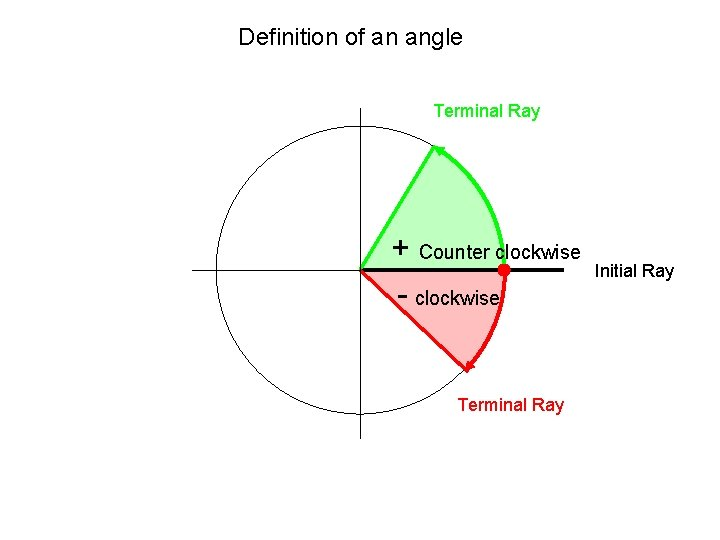Definition of an angle Terminal Ray + Counter clockwise - clockwise Terminal Ray Initial Ray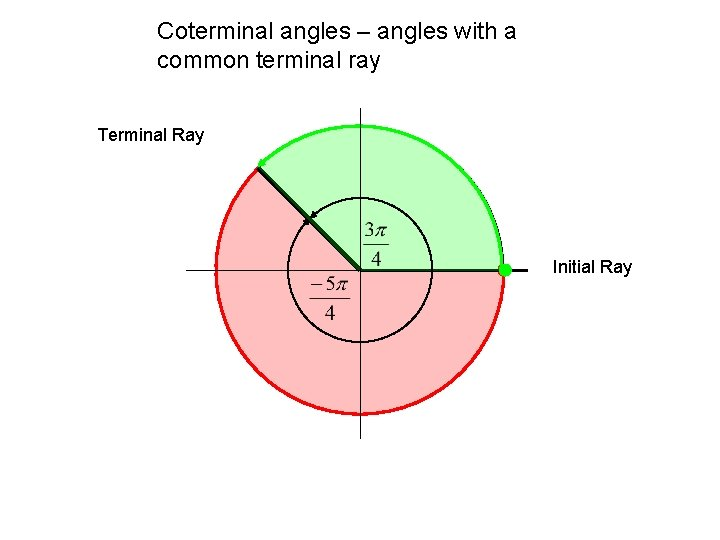Coterminal angles – angles with a common terminal ray Terminal Ray Initial RayCoterminal angles – angles with a common terminal ray Terminal Ray Initial Ray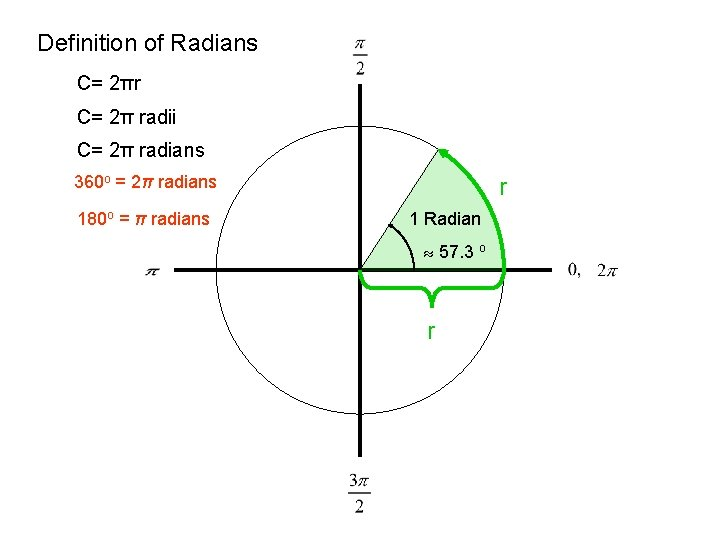Definition of Radians C= 2πr C= 2π radii C= 2π radians 360 o = 2π radians 180 o = π radians r 1 Radian 57. 3 o r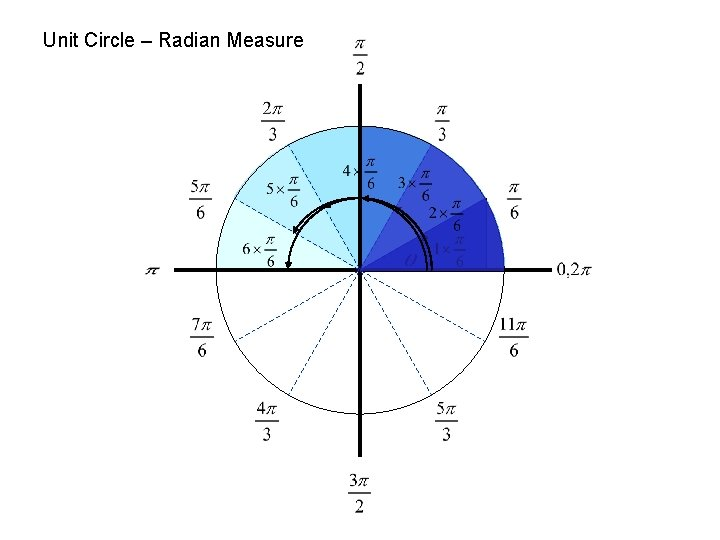Unit Circle – Radian Measure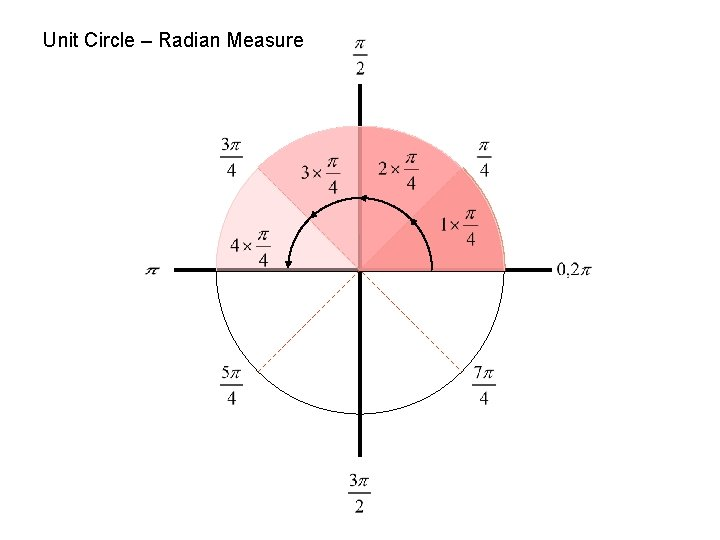Unit Circle – Radian MeasureUnit Circle – Radian Measure DegreesConverting Degrees ↔ Radians Converts degrees to Radians Recall Converts Radians to degrees more examples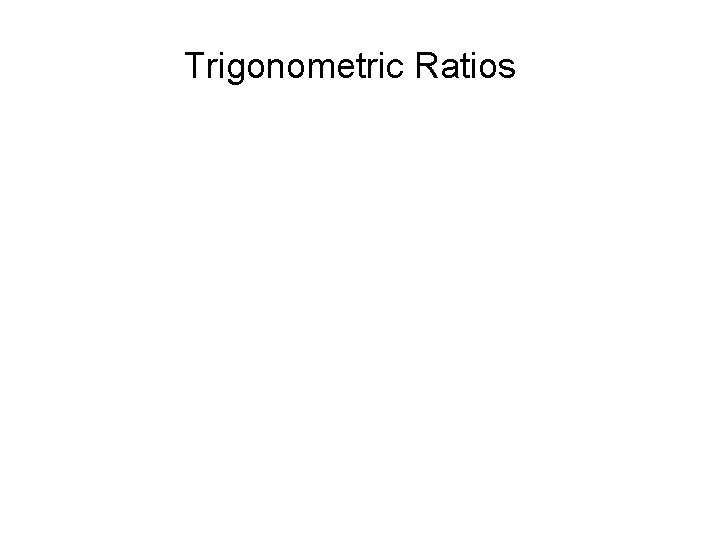Trigonometric Ratios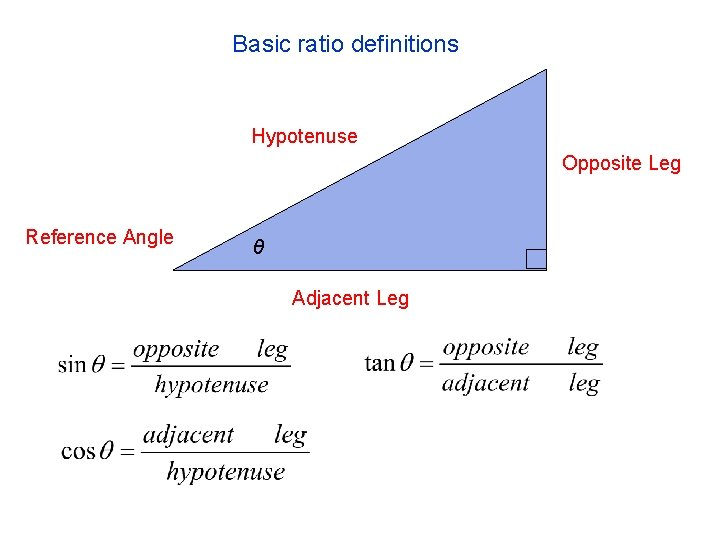Basic ratio definitions Hypotenuse Opposite Leg Reference Angle θ Adjacent LegCircle Trigonometry Definitions = s u i r d Ra Adjacent Leg = x reciprocal functions (x, y) Opposite Leg = y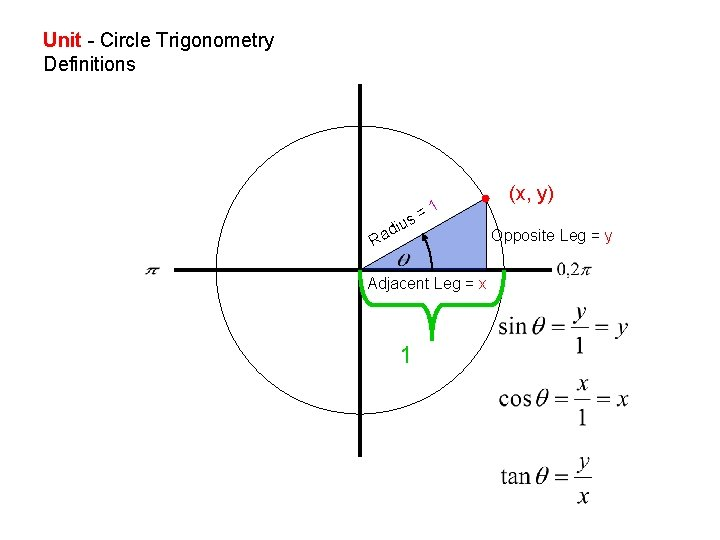Unit - Circle Trigonometry Definitions s iu ad =1 R Adjacent Leg = x 1 (x, y) Opposite Leg = yUnit Circle – Trig Ratios (-, +) (-, -) Skip π/4’s sin (+, +) (+, -) Reference Angles cos tan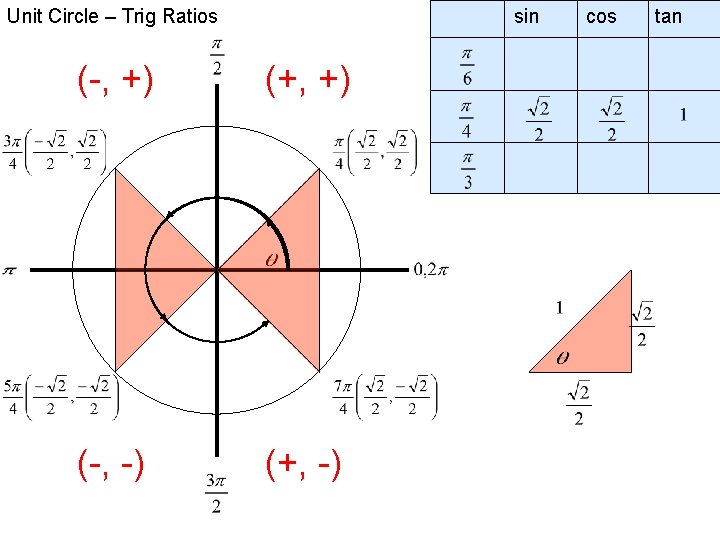Unit Circle – Trig Ratios sin (-, +) (+, +) (-, -) (+, -) cos tanUnit Circle – Trig Ratios (-, +) sin cos tan (+, +) (0 , 1) Quadrant Angles (-1, 0) (1, 0) 0 /2π (0, -1) (-, -) View π/4’s (+, -) sin cos tan 0 1 0 Ø 0 -1 0 Ø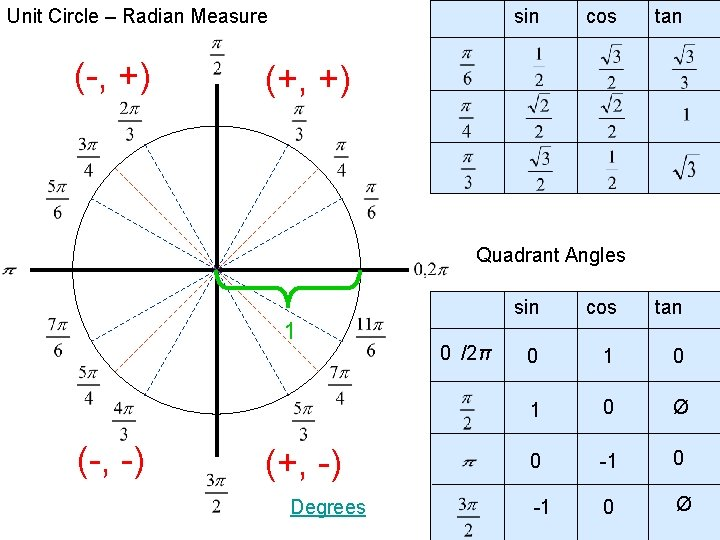Unit Circle – Radian Measure (-, +) sin cos tan (+, +) Quadrant Angles 1 (-, -) (+, -) Degrees 0 /2π sin cos tan 0 1 0 Ø 0 -1 0 ØA unit circle is a circle with a radius of 1 unit. For every point P(x, y) on the unit circle, the value of r is 1. Therefore, for an angle θ in the standard position: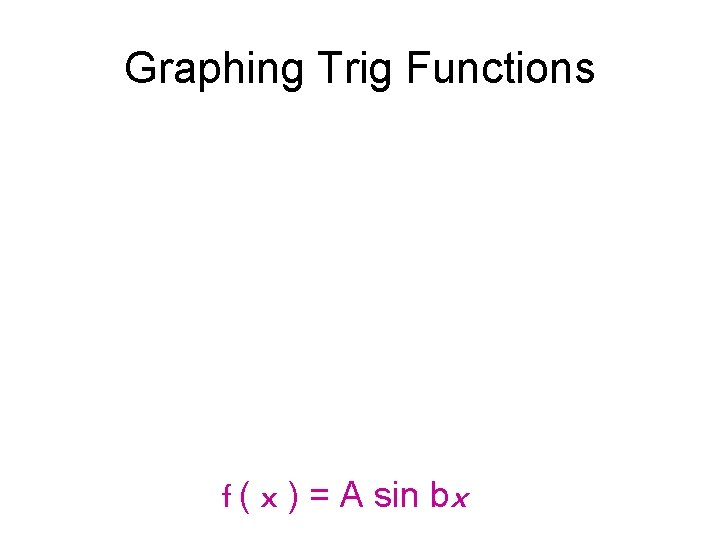Graphing Trig Functions f ( x ) = A sin bx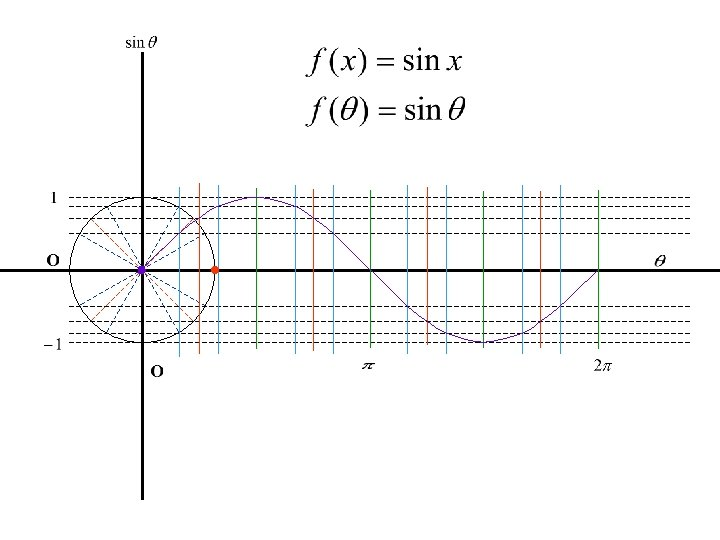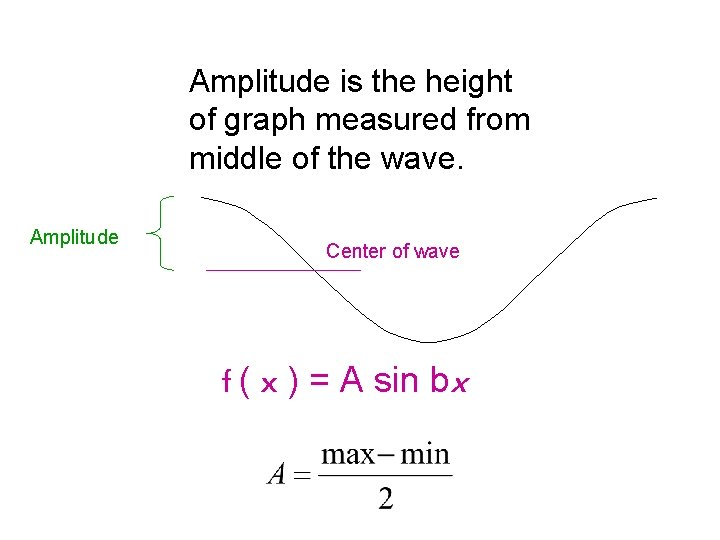Amplitude is the height of graph measured from middle of the wave. Amplitude Center of wave f ( x ) = A sin bxf ( x ) = cos x A = ½ , half as tallf ( x ) = sin x A = 2, twice as tall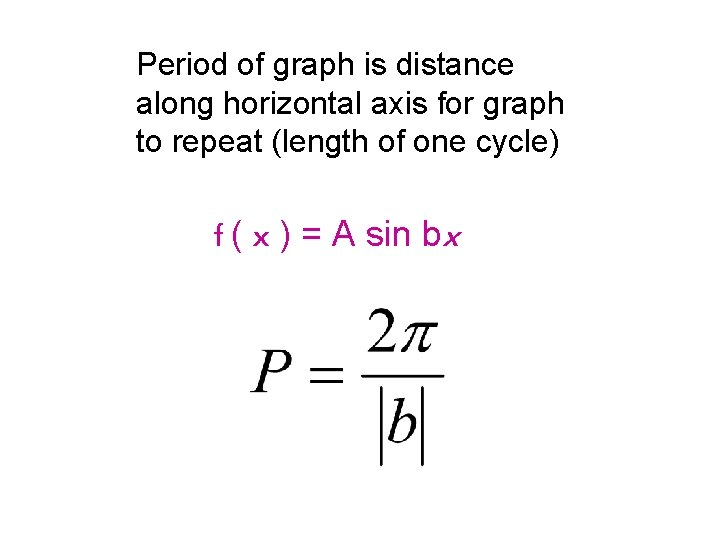Period of graph is distance along horizontal axis for graph to repeat (length of one cycle) f ( x ) = A sin bx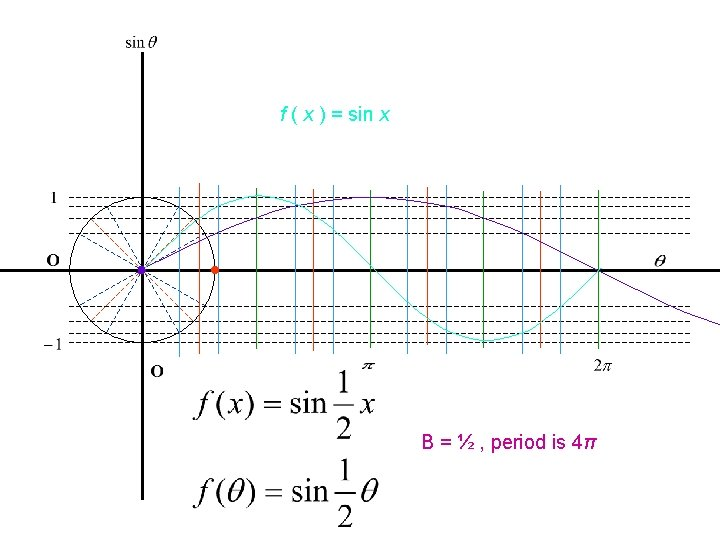f ( x ) = sin x B = ½ , period is 4πf ( x ) = cos x B = 2, period is π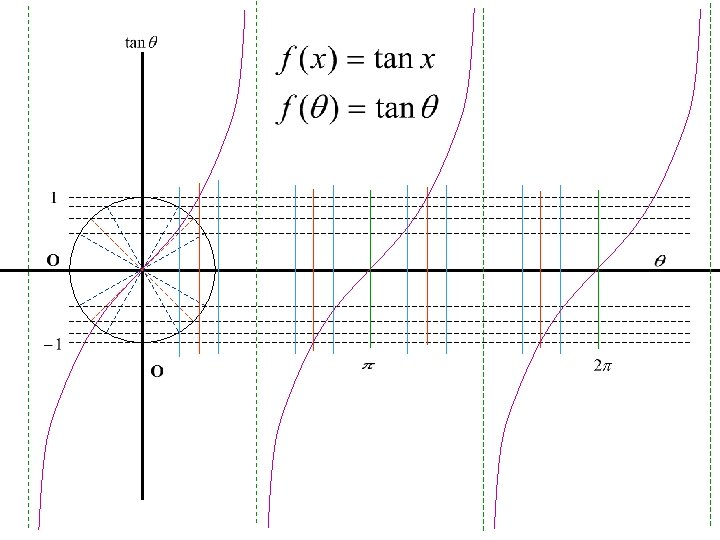Trigonometry Hipparchus, Menelaus, Ptolemy Special The Pythagoreans Graphs Right Triangles Rene’ Des. Cartes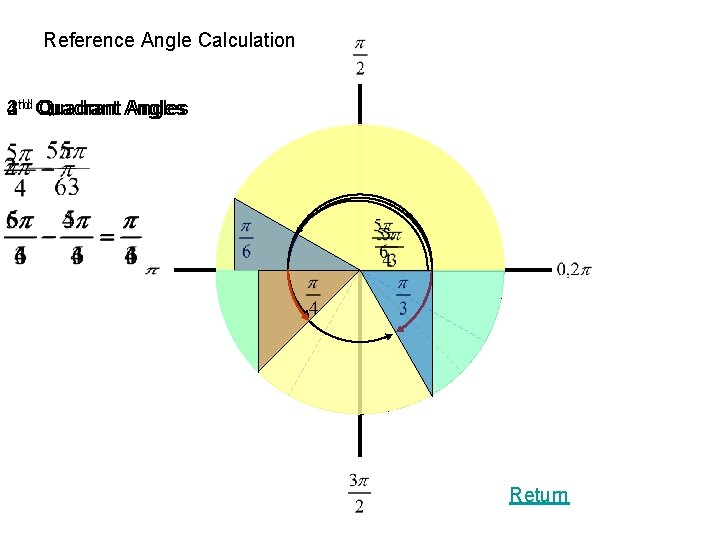Reference Angle Calculation nd Quadrant rd 4 th 2 3 Quadrant Angles Return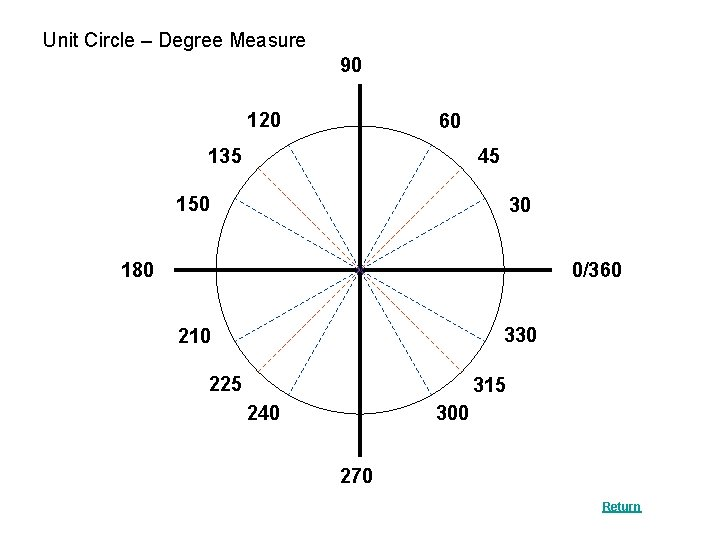Unit Circle – Degree Measure 90 120 60 45 135 150 30 180 0/360 330 210 225 315 300 240 270 Return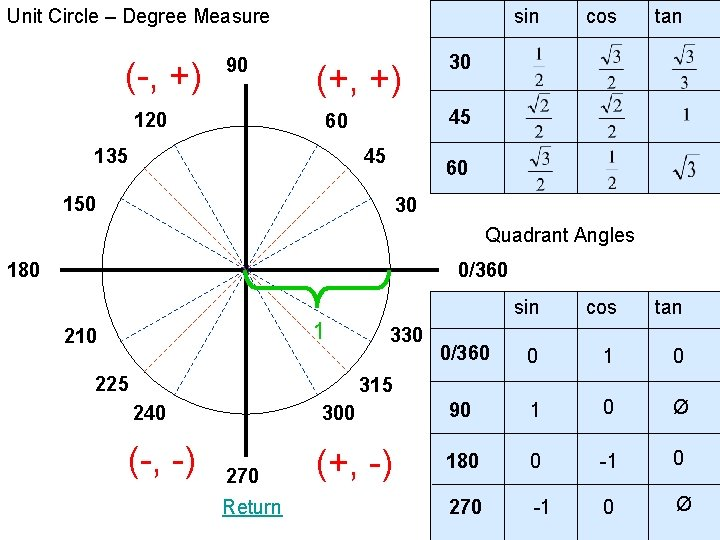Unit Circle – Degree Measure (-, +) 90 120 sin (+, +) tan 30 45 60 45 135 cos 60 150 30 Quadrant Angles 180 0/360 1 210 225 330 sin cos tan 0/360 0 1 0 315 240 300 90 1 0 Ø (-, -) (+, -) 180 0 -1 0 270 -1 0 Ø 270 Return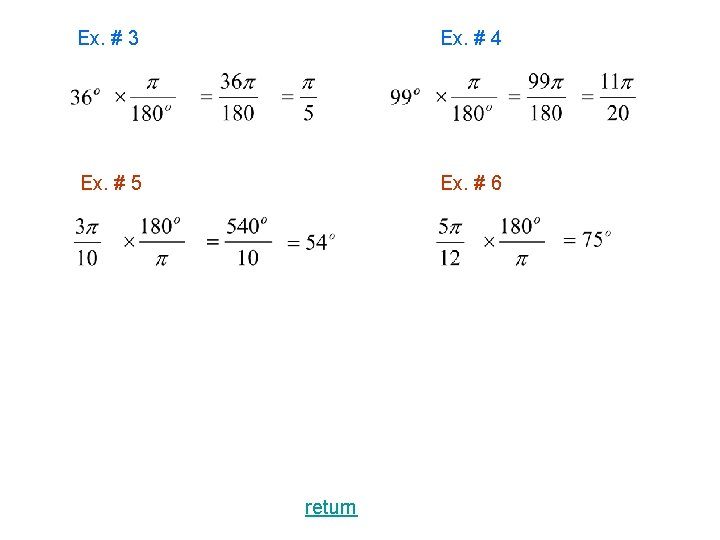Ex. # 3 Ex. # 4 Ex. # 5 Ex. # 6 returnCircle Trigonometry Definitions – Reciprocal Functions = s u i r d Ra Adjacent Leg = x return (x, y) Opposite Leg = y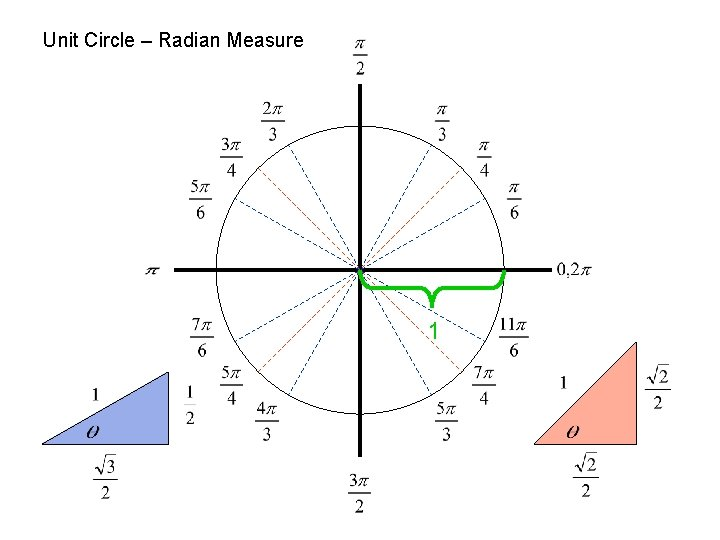Unit Circle – Radian Measure 1